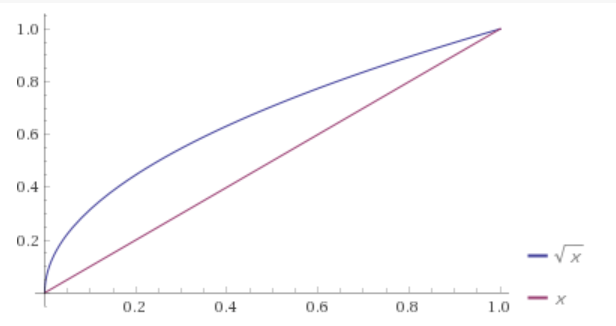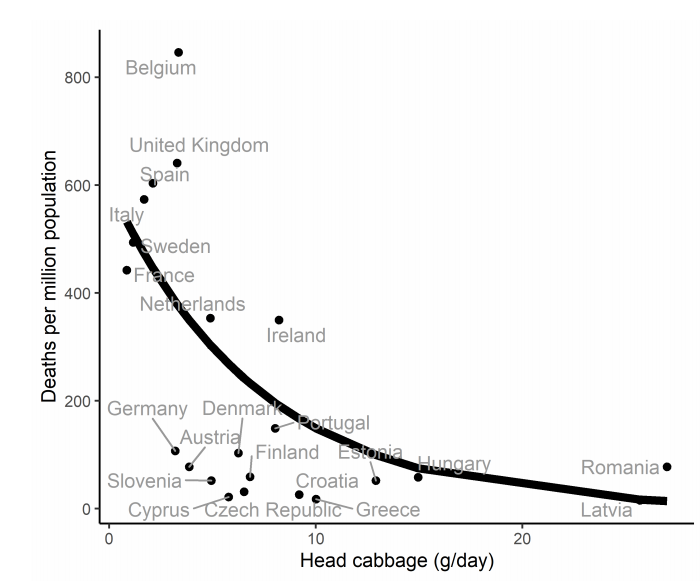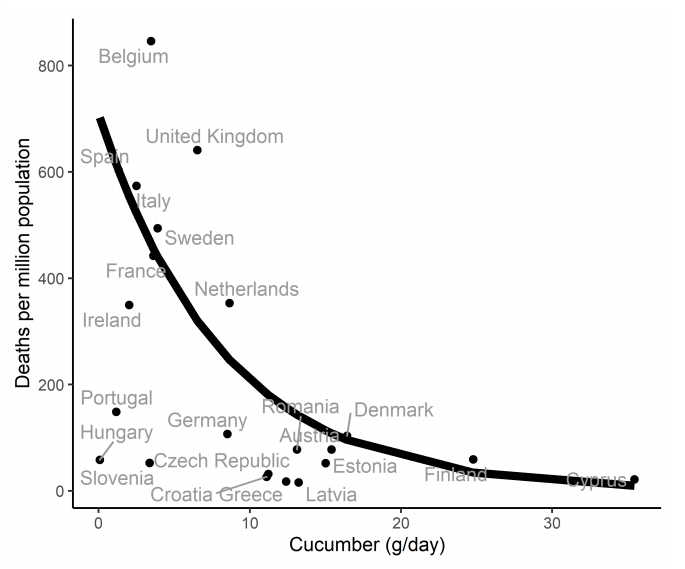## Thursday, July 30, 2020

### Seemingly simple but tricky NLP

People, mostly students, send me a lot of models for me to debug. Usually, I put them aside. A day has only 24 hours. Besides, students should discuss their problems with their teacher or supervisor instead of with me. But, here is one that is a bit more interesting. I reduced the model to its essence:

NLP Model
\begin{align}\min &\sum_{i,j} \color{darkblue} c_{i,j} \sqrt{\color{darkred}x_{i,j}}\\ & \sum_j \color{darkred}x_{i,j} = 1 && \forall i \\& \sum_i \color{darkred}x_{i,j} = 1 && \forall j \\ & \color{darkred}x_{i,j} \in [0,1] \end{align}

There are quite a few complications associated with this model:
• $$f(x)=\sqrt{x}$$ is not defined for $$x\lt 0$$,
• the derivative $$f'$$ can only be evaluated for $$x\gt 0$$ and it is very large for small $$x$$,
• if there is a positive $$c_{i,j} \gt 0$$, the objective function is non-convex.

Let's see what kind of problems and results we can encounter in practice.

#### Data

The random data set I used looks like:

----     19 PARAMETER c  cost coefficients

j1          j2          j3          j4          j5          j6          j7          j8          j9         j10

i1        1.717       8.433       5.504       3.011       2.922       2.241       3.498       8.563       0.671       5.002
i2        9.981       5.787       9.911       7.623       1.307       6.397       1.595       2.501       6.689       4.354
i3        3.597       3.514       1.315       1.501       5.891       8.309       2.308       6.657       7.759       3.037
i4        1.105       5.024       1.602       8.725       2.651       2.858       5.940       7.227       6.282       4.638
i5        4.133       1.177       3.142       0.466       3.386       1.821       6.457       5.607       7.700       2.978
i6        6.611       7.558       6.274       2.839       0.864       1.025       6.413       5.453       0.315       7.924
i7        0.728       1.757       5.256       7.502       1.781       0.341       5.851       6.212       3.894       3.587
i8        2.430       2.464       1.305       9.334       3.799       7.834       3.000       1.255       7.489       0.692
i9        2.020       0.051       2.696       4.999       1.513       1.742       3.306       3.169       3.221       9.640
i10       9.936       3.699       3.729       7.720       3.967       9.131       1.196       7.355       0.554       5.763


#### Global optimal solution

We can derive the optimal solution of the original problem NLP. When we look at the plot of $$f(x)=\sqrt{x}$$ against $$g(x)=x$$, we see that there is no good reason to be between 0 and 1:So actually we can solve the model as a linear assignment problem:

LP Model
\begin{align}\min &\sum_{i,j} \color{darkblue} c_{i,j} \color{darkred}x_{i,j}\\ & \sum_j \color{darkred}x_{i,j} = 1 && \forall i \\& \sum_i \color{darkred}x_{i,j} = 1 && \forall j \\ & \color{darkred}x_{i,j} \in [0,1] \end{align}

When we solve this problem with our data set we see:

----     32 **** LP solution ****
VARIABLE z.L                   =        9.202  objective

----     32 VARIABLE x.L

j1          j2          j3          j4          j5          j6          j7          j8          j9         j10

i1                                                                                                        1.000
i2                                                                                            1.000
i3                                1.000
i4        1.000
i5                                            1.000
i6                                                        1.000
i7                                                                    1.000
i8                                                                                                                    1.000
i9                    1.000
i10                                                                               1.000


As is to be expected when solving a pure linear assignment problem, the solution is automatically integer-valued.

A conclusion is that we just converted a very simple LP model into a very difficult NLP problem with all kinds of complications.

#### Reformulation

Many NLP algorithms employ tolerances such that $$x$$ values may be slightly outside their bounds. Also, values $$x_{i,j}=0$$ may lead to problems when trying to form gradients. Indeed, when we feed the model as is to IPOPT we see:

               S O L V E      S U M M A R Y

MODEL   m                   OBJECTIVE  z
TYPE    NLP                 DIRECTION  MINIMIZE
SOLVER  IPOPT               FROM LINE  28

**** SOLVER STATUS     4 Terminated By Solver
**** MODEL STATUS      7 Feasible Solution
**** OBJECTIVE VALUE              127.9104

RESOURCE USAGE, LIMIT          1.046      1000.000
ITERATION COUNT, LIMIT       162    2000000000
EVALUATION ERRORS             95             0

COIN-OR Ipopt    30.3.0 rc5da09e Released Mar 06, 2020 WEI x86 64bit/MS Window

**** ERRORS/WARNINGS IN EQUATION obj
50 error(s): sqrt: FUNC DOMAIN: x < 0
1 warning(s): sqrt: GRAD SINGULAR: x tiny


In addition, the solver log is full of really scary messages:

Warning: Cutting back alpha due to evaluation error
WARNING: Problem in step computation; switching to emergency mode.
Restoration phase is called at point that is almost feasible,
with constraint violation 4.626299e-11. Abort.


As IPOPT is an interior point solver, one may think that it only looks at points strictly inside $0 \lt \ x_{i,j} \lt 1$ In this case, we should not see the above domain errors. However, IPOPT will widen the bounds first, so the feasible region formed by the bounds becomes something like $0 -\delta \lt \ x_{i,j} \lt 1+\delta$

I actually expected IPOPT to terminate earlier, as the default GAMS limit for domain errors is 0. Also, I don't understand the bookkeeping. We see that there are 95 evaluation errors. But when looking where they appear, we just have 50 evaluation errors in the sqrt function. This is probably a bug in the IPOPT GAMS link.

One approach is to use a small non-zero lower bound on all variables: $x_{i,j} \in [\varepsilon,1]$ The main disadvantage is that we exclude a solution with $$x_{i,j}=0$$. A different way to handle this it to change the square root function a bit: $$\sqrt{\varepsilon+x_{i,j}}$$. In the experiments below I use a slightly improved version $\sqrt{\varepsilon+x_{i,j}}-\sqrt{\varepsilon}$ with $$\varepsilon=0.001$$.

When we solve our reformulated NLP model with a global solver we see:

----     37 **** Global NLP solution (Couenne, Antigone) ****
VARIABLE z.L                   =        8.915  objective

----     37 VARIABLE x.L

j1          j2          j3          j4          j5          j6          j7          j8          j9         j10

i1                                                                                                        1.000
i2                                                                                            1.000
i3                                1.000
i4        1.000
i5                                            1.000
i6                                                        1.000
i7                                                                    1.000
i8                                                                                                                    1.000
i9                    1.000
i10                                                                               1.000


Our objective a little bit off (remember: we perturbed the objective a bit) but the solution is the same as we expected from the linear model.

Strangely the global solver Baron is producing a slightly worse solution:

----     37 **** Global NLP solution (Baron) ****
VARIABLE z.L                   =       13.205  objective

----     37 VARIABLE x.L

j1          j2          j3          j4          j5          j6          j7          j8          j9         j10

i1                                            1.000
i2                                                        1.000
i3                                1.000
i4        1.000
i5                    1.000
i6                                                                                                        1.000
i7                                                                    1.000
i8                                                                                                                    1.000
i9                                                                                            1.000
i10                                                                               1.000


It is always good to try different solvers!

#### Local solvers

As the square root functions make the problem non-convex, we can expect local solutions. The local NLP solver MINOS produces an interesting solution:

----     37 **** Local NLP solution (Minos) ****
VARIABLE z.L                   =       44.343  objective

----     37 VARIABLE x.L

j1          j2          j3          j4          j5          j6          j7          j8          j9         j10

i1                                                                                                                    1.000
i2                                                                                                        1.000
i3                                                                                            1.000
i4                                                                                1.000
i5                                                                    1.000
i6                                                        1.000
i7                                            1.000
i8                                1.000
i9                    1.000
i10       1.000


The solver IPOPT still has problems with this model:

----     37 **** Local NLP solution (Ipopt) ****
VARIABLE z.L                   =       52.233  objective

----     37 VARIABLE x.L

j1          j2          j3          j4          j5          j6          j7          j8          j9         j10

i1        0.170                               0.208       0.086       0.147       0.120                   0.243       0.025
i2                    0.003                               0.228                   0.266       0.323                   0.179
i3        0.084       0.106       0.208       0.262                               0.176                               0.164
i4        0.238       0.059       0.242                   0.154       0.171                                           0.135
i5                    0.190       0.119       0.281       0.028       0.151                   0.082                   0.149
i6                                            0.222       0.184       0.197                   0.137       0.259
i7        0.206       0.180                               0.142       0.214                   0.010       0.115       0.132
i8        0.108       0.120       0.176                                           0.112       0.267                   0.216
i9        0.108       0.197       0.100       0.026       0.106       0.119       0.069       0.181       0.094
i10       0.086       0.144       0.155                   0.071                   0.257                   0.289

EXIT: Restoration Failed!
Final point is feasible: scaled constraint violation (3.33067e-16) is below tol (1e-08) and unscaled constraint violation (3.33067e-16) is below constr_viol_tol (0.0001).


I could fix this by making the tolerance $$\varepsilon$$ larger, from 0.001 to 0.01:

----     37 **** Local NLP solution (Ipopt, e=0.01) ****
VARIABLE z.L                   =        8.327  objective

----     37 VARIABLE x.L

j1          j2          j3          j4          j5          j6          j7          j8          j9         j10

i1                                                                                                        1.000
i2                                                                                            1.000
i3                                1.000
i4        1.000
i5                                            1.000
i6                                                        1.000
i7                                                                    1.000
i8                                                                                                                    1.000
i9                    1.000
i10                                                                               1.000


Some solvers are very careful not to try to evaluate functions outside the bounds. With $$\varepsilon=0$$, Conopt solves the model without issue (but to a location optimum), but mentions:

    C O N O P T 3   version 3.17K
Copyright (C)   ARKI Consulting and Development A/S
Bagsvaerdvej 246 A
DK-2880 Bagsvaerd, Denmark

The model has 101 variables and 21 constraints
with 301 Jacobian elements, 100 of which are nonlinear.
The Hessian of the Lagrangian has 100 elements on the diagonal,
0 elements below the diagonal, and 100 nonlinear variables.

** Warning **  The variance of the derivatives in the initial
point is large (= 14. ). A better initial
point, a better scaling, or better bounds on the
variables will probably help the optimization.


Interestingly Conopt is warning us about the variance of the derivatives. I would have expected it to complain about the size of the gradients. It is noted that the default initial point in GAMS is zero. To inspect the initial gradients we can look at the GAMS equation listing;

---- obj1  =E=

obj1..  - (17174713200)*x(i1,j1) - (84326670800)*x(i1,j2) - (55037535600)*x(i1,j3) - (30113790400)*x(i1,j4)

- (29221211700)*x(i1,j5) - (22405286700)*x(i1,j6) - (34983050400)*x(i1,j7) - (85627034700)*x(i1,j8) - (6711372300)*x(i1,j9)

- (50021066900)*x(i1,j10) - (99811762700)*x(i2,j1) - (57873337800)*x(i2,j2) - (99113303900)*x(i2,j3)

- (76225046700)*x(i2,j4) - (13069248300)*x(i2,j5) - (63971875900)*x(i2,j6) - (15951786400)*x(i2,j7) - (25008053300)*x(i2,j8)

- (66892860900)*x(i2,j9) - (43535638100)*x(i2,j10) - (35970026600)*x(i3,j1) - (35144136800)*x(i3,j2)

- (13149159000)*x(i3,j3) - (15010178800)*x(i3,j4) - (58911365000)*x(i3,j5) - (83089281200)*x(i3,j6) - (23081573800)*x(i3,j7)

- (66573446000)*x(i3,j8) - (77585760600)*x(i3,j9) - (30365847700)*x(i3,j10) - (11049229100)*x(i4,j1)

- (50238486600)*x(i4,j2) - (16017276200)*x(i4,j3) - (87246231100)*x(i4,j4) - (26511454500)*x(i4,j5) - (28581432200)*x(i4,j6)

- (59395592200)*x(i4,j7) - (72271907100)*x(i4,j8) - (62824867700)*x(i4,j9) - (46379786500)*x(i4,j10)

- (41330699400)*x(i5,j1) - (11769535700)*x(i5,j2) - (31421226700)*x(i5,j3) - (4655151400)*x(i5,j4) - (33855027200)*x(i5,j5)

- (18209959300)*x(i5,j6) - (64572712700)*x(i5,j7) - (56074554700)*x(i5,j8) - (76996172000)*x(i5,j9)

- (29780586400)*x(i5,j10) - (66110626100)*x(i6,j1) - (75582167400)*x(i6,j2) - (62744749900)*x(i6,j3)

- (28386419800)*x(i6,j4) - (8642462400)*x(i6,j5) - (10251466900)*x(i6,j6) - (64125115100)*x(i6,j7) - (54530949800)*x(i6,j8)

- (3152485200)*x(i6,j9) - (79236064200)*x(i6,j10) - (7276699800)*x(i7,j1) - (17566104900)*x(i7,j2) - (52563261300)*x(i7,j3)

- (75020766900)*x(i7,j4) - (17812371400)*x(i7,j5) - (3414098600)*x(i7,j6) - (58513117300)*x(i7,j7) - (62122998400)*x(i7,j8)

- (38936190000)*x(i7,j9) - (35871415300)*x(i7,j10) - (24303461700)*x(i8,j1) - (24642153900)*x(i8,j2)

- (13050280300)*x(i8,j3) - (93344972000)*x(i8,j4) - (37993790600)*x(i8,j5) - (78340046100)*x(i8,j6) - (30003425800)*x(i8,j7)

- (12548322200)*x(i8,j8) - (74887410500)*x(i8,j9) - (6923246300)*x(i8,j10) - (20201555700)*x(i9,j1) - (506585800)*x(i9,j2)

- (26961305200)*x(i9,j3) - (49985147500)*x(i9,j4) - (15128586900)*x(i9,j5) - (17416945500)*x(i9,j6) - (33063773400)*x(i9,j7)

- (31690605400)*x(i9,j8) - (32208695500)*x(i9,j9) - (96397664100)*x(i9,j10) - (99360220500)*x(i10,j1)

- (36990305500)*x(i10,j2) - (37288856700)*x(i10,j3) - (77197833000)*x(i10,j4) - (39668414200)*x(i10,j5)

- (91309632500)*x(i10,j6) - (11957773000)*x(i10,j7) - (73547888900)*x(i10,j8) - (5541847500)*x(i10,j9)

- (57629980500)*x(i10,j10) + z =E= 0 ; (LHS = 0)



This shows the linearized objective. The numbers in parentheses are gradients. We see that they are very large (this is how GAMS returns the gradient at zero; instead of returning infinity a large number is returned). It also shows the variance Conopt is talking about.

#### Conclusion

Just by making the assignment problem a little bit nonlinear, we can get into major problems.

## Tuesday, July 21, 2020

### Cabbage against covid

Some statistics in action :"After adjusting for potential confounders, for each g/day increase in consumption of head cabbage of the country, the mortality risk for COVID-19 decreases by 13.6 %."

But wait, in case you don't like cabbage, we have some cucumber on offer: "For each g/day increase in consumption of cucumber, the mortality risk decreases by 15.7%."Lettuce or broccoli is not that good: "Consumption of lettuce and broccoli showed the opposite pattern, i.e. a higher consumption was associated with a higher COVID-19 mortality."

#### References

1. Susana C Fonseca, Ioar Rivas, Dora Romaguera, Marcos Quijal-Zamorano, Wienczyslawa Czarlewski, Alain Vidal, Joao A Fonseca, Joan Ballester, Josep M Anto, Xavier Basagana, Luis M Cunha, Jean Bousquet, Association between consumption of vegetables and COVID-19 mortality at a country level in Europe, preprint, https://www.medrxiv.org/content/10.1101/2020.07.17.20155846v1